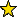# ModuleDeformations -- versal deformations of modules on hypersurfaces

## Description

Given a regular element $f$ in $R$, $R$ a regular ring, a matrix factorisation of $f$ is a pair $(A,B)$ of square matrices of the same size over $S$ such that $AB = fI = BA$, where $I$ is the identity matrix of the same dimension. Matrix factorisations of $f$ are equivalent to maximal Cohen-Macaulay (MCM) modules on the hypersurface defined by $f$: given a matrix factorisation $(A,B)$ of $f$, the cokernel of $A$ is an MCM-module over $S/(f)$, and all MCM-modules over $S/(f)$ arise in this way.

Let $X$ be a germ of a hypersurface at a point 0 and $M_0$ be a maximal Cohen-Macaulay module over $X$. Let $Y \rightarrow{} \Sigma$ a flat map of germs of affine varieties at 0 whose fibre over 0 of $\Sigma$ is isomorphic to $X$. A deformation of $M_0$ (in the deformation theory defined by the map $Y \rightarrow{} \Sigma$) is a pair $(S,M)$ with $S$ a germ equipped with a map $S \rightarrow{} \Sigma$ and $M$ a module over $S \times_\Sigma Y$ flat over $S$, whose restriction to $0 \times_\Sigma X$ is isomorphic to $M_0$.

A deformation $(T,M')$ is induced from$(S,M)$ if there exists a map $\phi:T \rightarrow{} S$ commuting with the maps $T \rightarrow{} \Sigma$ and $S \rightarrow{} \Sigma$ and such that $M'$ is isomorphic to $(\phi \times_\Sigma id_Y)*M$.

The deformation $(S,M)$ is versal if any deformation of $M_0$ is induced from $(S,M)$.

In this package, a germ is represented as the coordinate ring R of an affine variety and the distinguished point 0 of a germ is assumed to be the ideal generated by the variables of R. It is an error if the ideal generated by the variables of R is not prime, e.g. if it is all of R.

This package contains two entry points. One interface may be used only in the absolute case, where $\Sigma$ is a reduced point, and the module $M_0$ is deformed over $Y$ only. The other interface may be used when $\Sigma$ is the base space of a nontrivial deformation of $X$ and $Y$ is the total space of the deformation. In such a case, $M_0$ is deformed also along the given deformation of $X$.

The implementation has been designed to take advantage of graded structure when available. Thus the user is highly recommended to assign degrees to the inputs so as to make the rings, homomorphism, and deformation module homogeneous when possible. Doing so may greatly improve performance.

## CertificationVersion 1.0 of this package was accepted for publication in volume 2 of The Journal of Software for Algebra and Geometry: Macaulay2 on 2010-04-17, in the article Deformations of Matrix Factorisations with Macaulay2. That version can be obtained from the journal or from the Macaulay2 source code repository.

## Version

This documentation describes version 1.0 of ModuleDeformations.

## Source code

The source code from which this documentation is derived is in the file ModuleDeformations.m2.

## For the programmer

The object ModuleDeformations is .